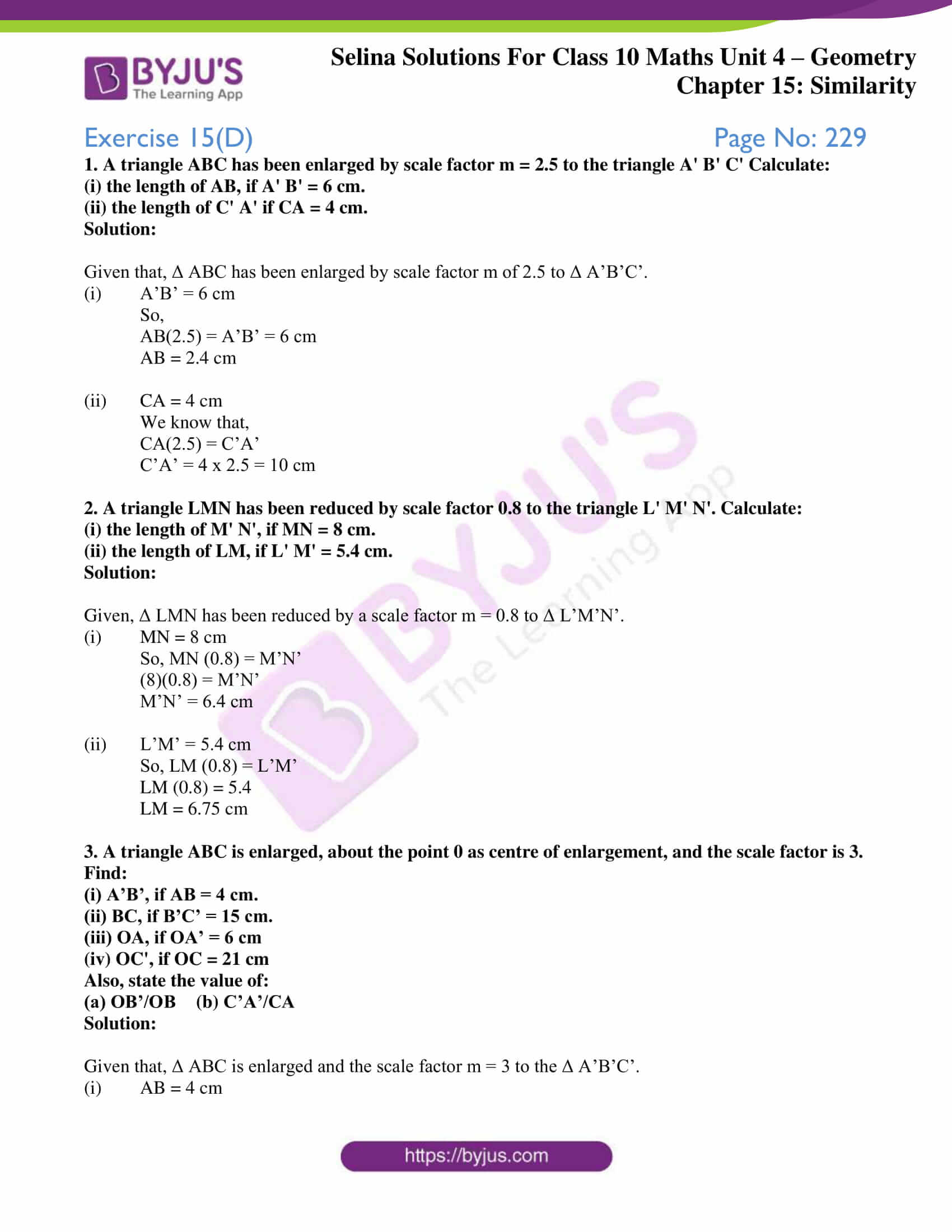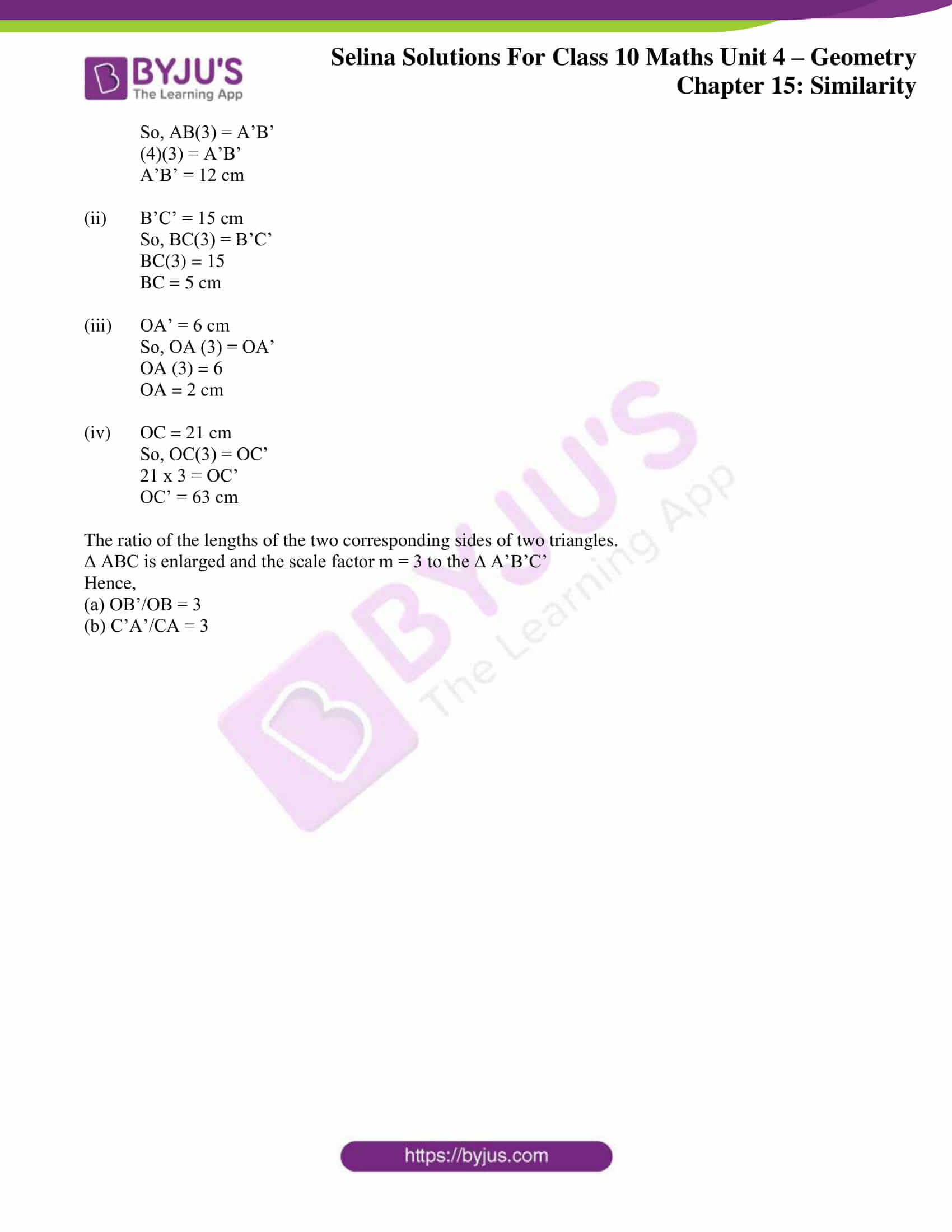# Selina Solutions Concise Maths Class 10 Chapter 15 Similarity (With Applications to Maps and Models) Exercise 15(D)

This exercise majorly contains problems based on similarity as a size transformation and applications to maps and models. Students needing any guidance in understanding concepts can make use of the Selina Solutions for Class 10 Maths which is prepared by subject experts at BYJU’S. In addition, all the solutions are according to the latest ICSE patterns. Selina Solutions Concise Maths Class 10 Chapter 15 Similarity (With Applications to Maps and Models) Exercise 15(D) can be accessed in PDF format, from the links provided below.

## Selina Solutions Concise Maths Class 10 Chapter 15 Similarity (With Applications to Maps and Models) Exercise 15(D) Download PDF### Access other exercises of Selina Solutions Concise Maths Class 10 Chapter 15 Similarity (With Applications to Maps and Models)

Exercise 15(A) Solutions

Exercise 15(B) Solutions

Exercise 15(C) Solutions

Exercise 15(E) Solutions

## Access Selina Solutions Concise Maths Class 10 Chapter 15 Similarity (With Applications to Maps and Models)

#### Exercise 15(D) Page No: 229

1. A triangle ABC has been enlarged by scale factor m = 2.5 to the triangle A’ B’ C’ Calculate:

(i) the length of AB, if A’ B’ = 6 cm.

(ii) the length of C’ A’ if CA = 4 cm.

Solution:

Given that, Δ ABC has been enlarged by scale factor m of 2.5 to Δ A’B’C’.

(i) A’B’ = 6 cm

So,

AB(2.5) = A’B’ = 6 cm

AB = 2.4 cm

(ii) CA = 4 cm

We know that,

CA(2.5) = C’A’

C’A’ = 4 x 2.5 = 10 cm

2. A triangle LMN has been reduced by scale factor 0.8 to the triangle L’ M’ N’. Calculate:

(i) the length of M’ N’, if MN = 8 cm.

(ii) the length of LM, if L’ M’ = 5.4 cm.

Solution:

Given, Δ LMN has been reduced by a scale factor m = 0.8 to Δ L’M’N’.

(i) MN = 8 cm

So, MN (0.8) = M’N’

(8)(0.8) = M’N’

M’N’ = 6.4 cm

(ii) L’M’ = 5.4 cm

So, LM (0.8) = L’M’

LM (0.8) = 5.4

LM = 6.75 cm

3. A triangle ABC is enlarged, about the point 0 as centre of enlargement, and the scale factor is 3. Find:

(i) A’B’, if AB = 4 cm.

(ii) BC, if B’C’ = 15 cm.

(iii) OA, if OA’ = 6 cm

(iv) OC’, if OC = 21 cm

Also, state the value of:

(a) OB’/OB (b) C’A’/CA

Solution:

Given that, Δ ABC is enlarged and the scale factor m = 3 to the Δ A’B’C’.

(i) AB = 4 cm

So, AB(3) = A’B’

(4)(3) = A’B’

A’B’ = 12 cm

(ii) B’C’ = 15 cm

So, BC(3) = B’C’

BC(3) = 15

BC = 5 cm

(iii) OA’ = 6 cm

So, OA (3) = OA’

OA (3) = 6

OA = 2 cm

(iv) OC = 21 cm

So, OC(3) = OC’

21 x 3 = OC’

OC’ = 63 cm

The ratio of the lengths of the two corresponding sides of two triangles.

Δ ABC is enlarged and the scale factor m = 3 to the Δ A’B’C’

Hence,

(a) OB’/OB = 3

(b) C’A’/CA = 3